# Exploring Data Distribution | Set 1

Whenever we work in data science and machine learning, our approach of handling the data and finding something useful out of it is based on the distribution of the data.
Distribution means that how data can be present in different possible ways, the percentage of specific data, identifying the outliers. So, data distribution is the way of using graphical methods to organize and display useful information.

Terms related to Exploration of Data Distribution

```-> Boxplot
-> Frequency Table
-> Histogram
-> Density Plot
```
• Boxplot : It is based on the percentiles of the data as shown in the figure below. The top and bottom of the boxplot are 75th and 25th percentile of the data. The extended lines are known as whiskers that includes the range of rest of the data.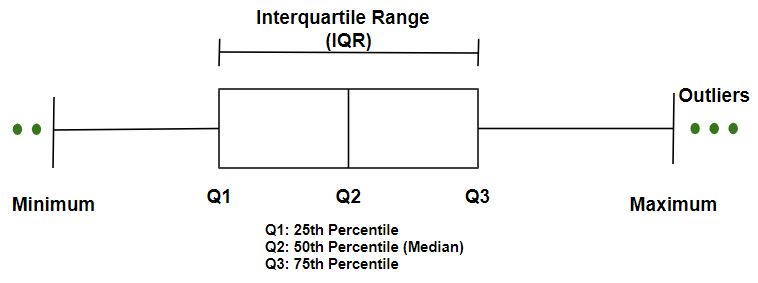To get the link to `csv `file used, click here.

 `import` `numpy as np ` `import` `pandas as pd ` `import` `seaborn as sns ` `import` `matplotlib.pyplot as plt `

 `data ``=` `pd.read_csv(``"../data/state.csv"``) ` ` `  `# Adding a new column with derived data  ` `data[``'PopulationInMillions'``] ``=` `data[``'Population'``]``/``1000000` ` `  `print` `(data.head(``10``)) `

Output :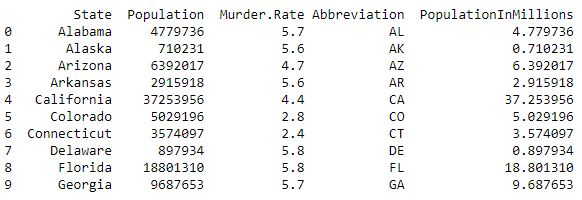Code #3 : BoxPlot

 `# BoxPlot Population In Millions ` `fig, ax1 ``=` `plt.subplots() ` `fig.set_size_inches(``9``,  ``15``) ` ` `  `ax1 ``=` `sns.boxplot(x ``=` `data.PopulationInMillions, orient ``=``"v"``) ` `ax1.set_ylabel(``"Population by State in Millions"``, fontsize ``=` `15``) ` `ax1.set_title(``"Population - BoxPlot"``, fontsize ``=` `20``) `

Output :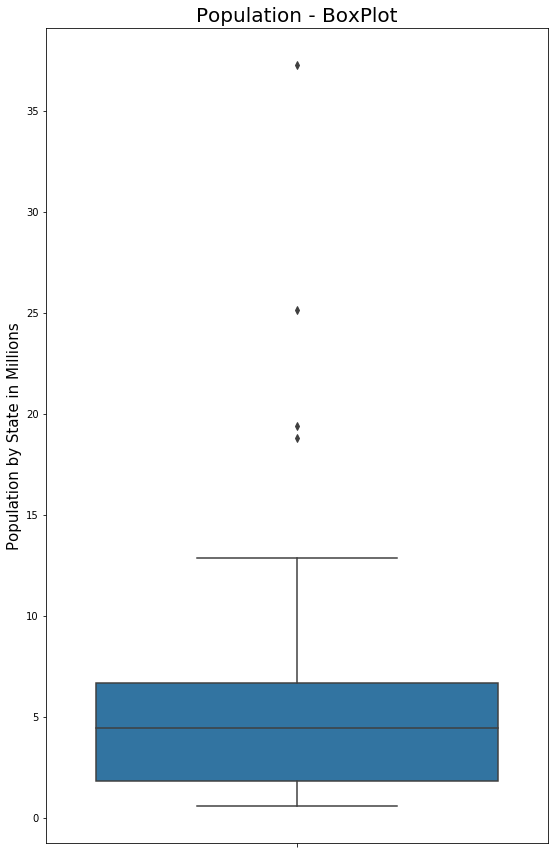• Frequency Table : It is a tool to distribute the data into equally spaced ranges, segments and tells us how many values fall in each segment.

Code #1: Adding a column to perform crosstab and groupby functionality.

 `# Perform the binning action, the bins have been ` `# chosen to accentuate the output for the Frequency Table ` ` `  `data[``'PopulationInMillionsBins'``] ``=` `pd.cut( ` `    ``data.PopulationInMillions, bins ``=``[``0``, ``1``, ``2``, ``5``, ``8``, ``12``, ``15``, ``20``, ``50``]) ` ` `  `print` `(data.head(``10``)) `

Output :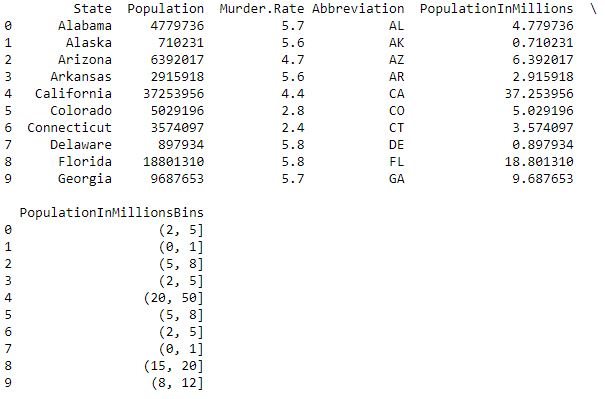Code #2: Cross Tab – a type of Frequency Table

 `# Cross Tab - a type of Frequency Table ` ` `  `pd.crosstab(data.PopulationInMillionsBins, data.Abbreviation, margins ``=` `True``) `

Output :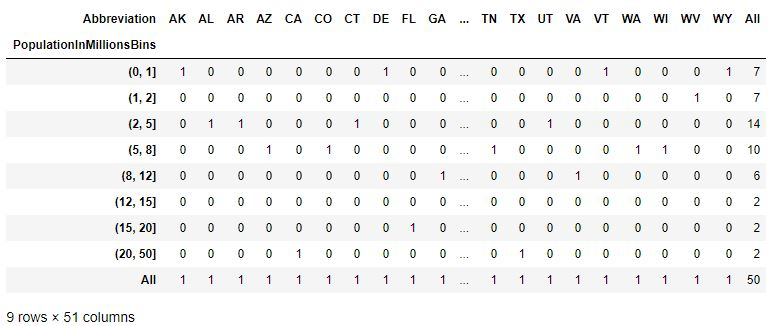Code #3: GroupBy – a type of Frequency Table

 `# Groupby - a type of Frequency Table ` ` `  `data.groupby(data.PopulationInMillionsBins)[``'Abbreviation'``].``apply``(``', '``.join) `

Output :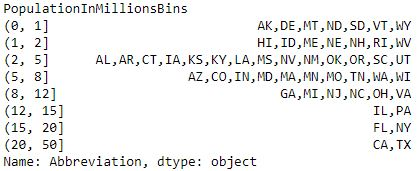My Personal Notes arrow_drop_upIf you like GeeksforGeeks and would like to contribute, you can also write an article using contribute.geeksforgeeks.org or mail your article to contribute@geeksforgeeks.org. See your article appearing on the GeeksforGeeks main page and help other Geeks.

Please Improve this article if you find anything incorrect by clicking on the "Improve Article" button below.